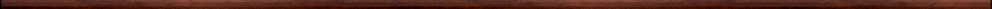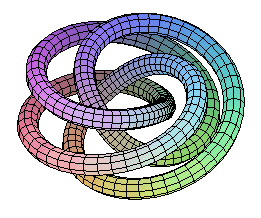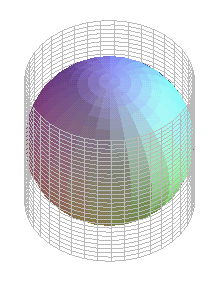Maple worksheets on geometryIntroductory, general and miscellaneous worksheets:

They are all compatible with Classic Worksheet Maple 10.Polygons - polygon.mws

• Plotting polygons
• Rotating polygon animation - 1 colour
• A polygon subdivided into different coloured triangles
• Rotating polygon animation - 2 colours

An introduction to 3 dimensional images - graph3D.mws

• The 3-dimensional coordinate system
• Regular polyhedra via the plottools package.
• Regular polyhedra via the geom3d package.
• Families of polyhedra
• spacecurve and tubeplot
• Torus knots

Volumes of solids - vol3D.mws

• Some standard solids.
• A procedure for constructing a prism: prism.
• A procedure for constructing a pyramid: pyramid.
• The volume of a prism.
• The volume of a cylinder.
• The volume of a pyramid 1 . . sheering transformation.
• The volume of a pyramid 2 . . dissection of a cuboid.
• The volume of a cone.
• The surface area of a sphere.
• The volume of a sphere.

Calculating pi - calcpi.mws

• Calculating Pi using regular polygons with a progressively larger number of sidesTop of page

Main index